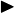# Parametric vs Non-parametric algorithms

When browsing through tutorials and reading books, sometimes we see the terms ‘Parametric‘ and ‘Non-parametric‘ algorithms. The authors of the tutorials and books suggest when we should use this type of algorithms and when to use the other, but how do we differentiate between these two? This blog post will show us how!

Let me take a subtle note before we begin, hopefully, this will not drag your mood down, but the separating line of Parametric and Non-parametric is somehow vague, meaning the discrimination is a bit ambiguous. Nevertheless, it is just “a bit ambiguous”, not “very ambiguous”, so don’t be depressed, ok?

PropertiesThe most important factor to determine if an algorithm is parametric or not depends on its nature and assumptions: a parametric algorithm has some assumptions about the underlying characteristics of the function it estimates, while a non-parametric algorithm does not.

E.g.The second trait is: for parametric algorithms, the number of parameters is usually fixed, while for non-parametric algorithms, it can potentially grow to infinity, depending on the training data.

E.g.The third and also the last property is: The parameters of parametric models usually have their meaning (i.e. role) in explaining how the models work, while this is not true for non-parametric models.

E.g.

Examples

Some parametric algorithms are:

• Linear regression
• Logistic regression
• Gaussian Naive Bayes

Some non-parametric algorithms are:

• K-nearest neighbors
• Decision tree
• Deep neural networks
• Support vector machine
• Naive Bayes with Density estimation

Each type of algorithm has its own advantages and disadvantages. While we look at them from this angle, there are only 2 types, so the advantages of this type are the disadvantages of the other type and vice versa.

The parametric algorithms usually have below strengths:

• Simpler and more intuitive.
• Faster to train and give predictions.
• Require fewer data.
• Interpretable.

To the contrary, non-parametric algorithms thrive on:

• Have flexibility, more versatile as they do not make assumptions about data.
• Stronger performance if being provided enough data.

To sum up

In this blog post, we discussed the differences and how to differentiate parametric and non-parametric algorithms. In short, parametric algorithms (represented by Linear regression) make strong assumptions about the data, thus they require fewer data to train and lesser time to run. On the other hand, Non-parametric algorithms (e.g. Deep neural networks) do not assume prior knowledge of the data, which makes them more versatile.

References: